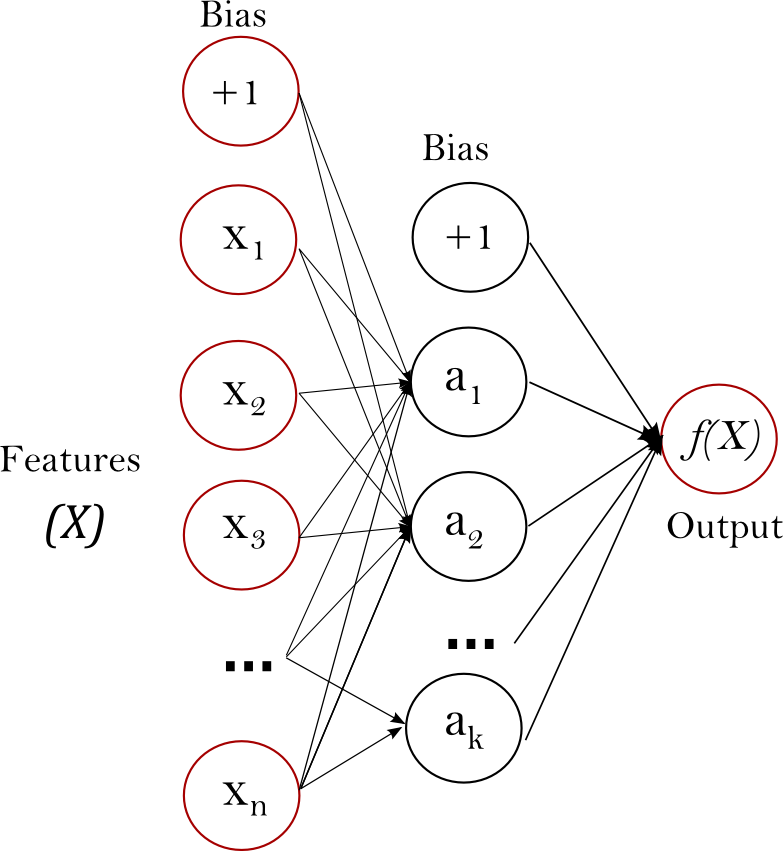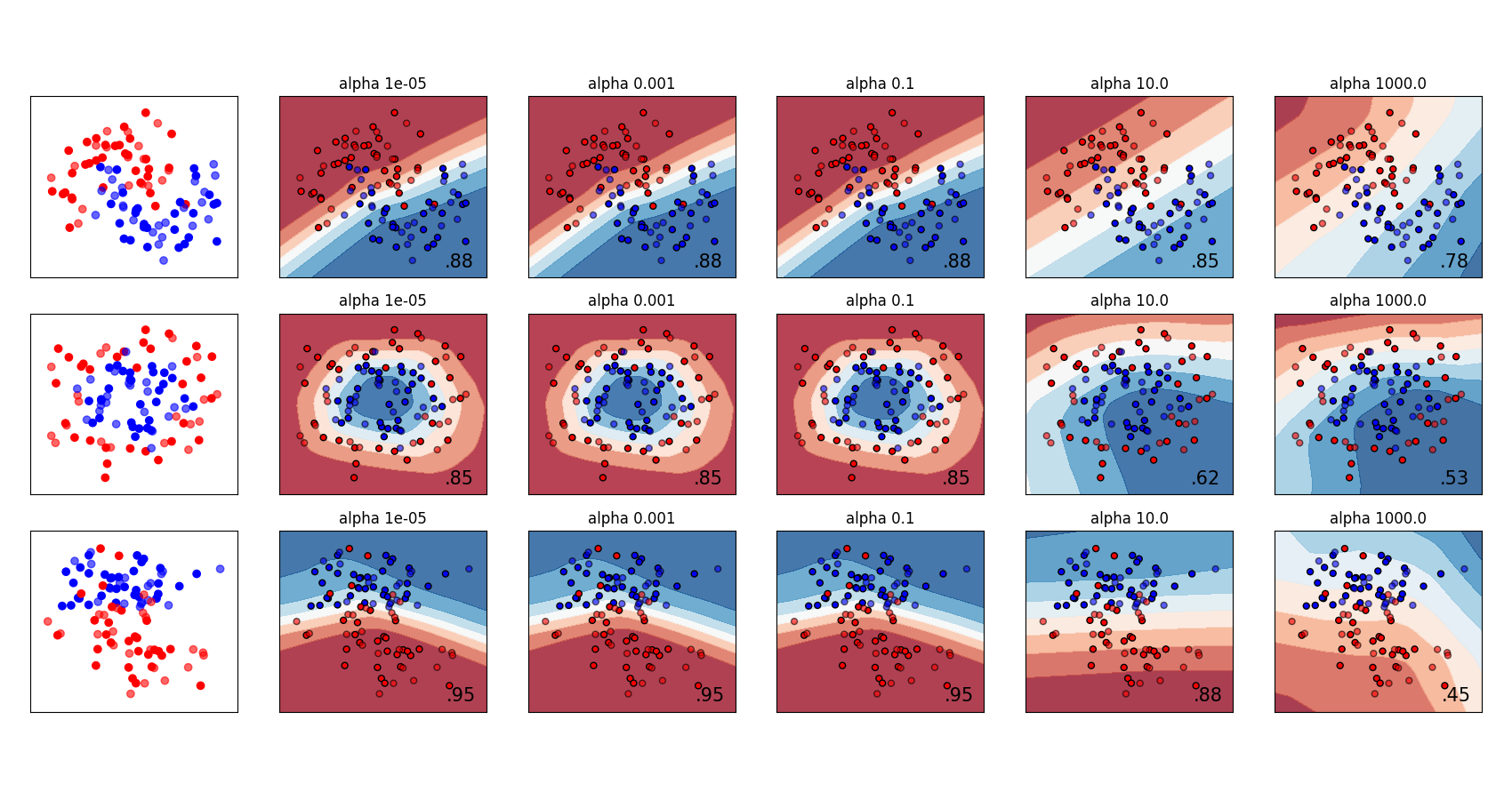# 1.17. 神经网络模型（有监督）

## 1.17.1. 多层感知器• 可以学习得到非线性模型。
• 使用partial_fit 可以学习得到实时模型（在线学习）。

• 具有隐藏层的 MLP 具有非凸的损失函数，它有不止一个的局部最小值。 因此不同的随机初始化权重会导致不同的验证集准确率。
• MLP 需要调试一些超参数，例如隐藏层神经元的数量、层数和迭代轮数。
• MLP 对特征归一化很敏感。

## 1.17.2. 分类

MLPClassifier 类实现了通过 Backpropagation 进行训练的多层感知器（MLP）算法。

MLP 在两个数组上进行训练：大小为 (n_samples, n_features) 的数组 X，用来储存表示训练样本的浮点型特征向量; 大小为 (n_samples,) 的数组 y，用来储存训练样本的目标值（类别标签）：

>>> from sklearn.neural_network import MLPClassifier
>>> X = [[0., 0.], [1., 1.]]
>>> y = [0, 1]
>>> clf = MLPClassifier(solver='lbfgs', alpha=1e-5,
...                     hidden_layer_sizes=(5, 2), random_state=1)
...
>>> clf.fit(X, y)
MLPClassifier(alpha=1e-05, hidden_layer_sizes=(5, 2), random_state=1,
solver='lbfgs')


>>> clf.predict([[2., 2.], [-1., -2.]])
array([1, 0])


MLP 可以为训练数据拟合一个非线性模型。 clf.coefs_ 包含了构成模型参数的权值矩阵：

>>> [coef.shape for coef in clf.coefs_]
[(2, 5), (5, 2), (2, 1)]


MLP 算法使用的是反向传播的方式。 更准确地说，它使用了某种形式的梯度下降来进行训练，其中的梯度是通过反向传播计算得到的。 对于分类问题而言，它最小化了交叉熵损失函数，为每个样本给出一个向量形式的概率估计>>> clf.predict_proba([[2., 2.], [1., 2.]])
array([[1.967...e-04, 9.998...-01],
[1.967...e-04, 9.998...-01]])


MLPClassifier 通过应用 Softmax 作为输出函数来支持多分类。

>>> X = [[0., 0.], [1., 1.]]
>>> y = [[0, 1], [1, 1]]
>>> clf = MLPClassifier(solver='lbfgs', alpha=1e-5,
...                     hidden_layer_sizes=(15,), random_state=1)
...
>>> clf.fit(X, y)
MLPClassifier(alpha=1e-05, hidden_layer_sizes=(15,), random_state=1,
solver='lbfgs')
>>> clf.predict([[1., 2.]])
array([[1, 1]])
>>> clf.predict([[0., 0.]])
array([[0, 1]])


## 1.17.3. 回归

MLPRegressor 类实现了多层感知器（MLP），它使用反向传播进行训练，输出层没有使用激活函数，也可以被看作是使用恒等函数（identity function）作为激活函数。 因此，它使用平方误差作为损失函数，输出是一组连续值。

MLPRegressor 还支持多输出回归，其中一个样本可以有多个目标值。

## 1.17.4. 正则化

MLPRegressor 类和 MLPClassifier 类都使用参数 alpha 作为正则化( L2 正则化)系数，正则化通过惩罚大数量级的权重值以避免过拟合问题。 下面的图表展示了不同的 alpha 值下的决策函数的变化。## 1.17.5. 算法L-BFGS 是一种粗略估计表示函数二阶偏导数的 Hessian 矩阵的求解器，它粗略估计 Hessian 矩阵的逆来进行参数更新。该实现使用 Scipy 版本的 L-BFGS

## 1.17.7. 数学公式MLP 根据特定问题使用不同的损失函数。 分类问题的损失函数的是交叉熵，在二分类情况中具体形式如下，## 1.17.8. 实用技巧

• 多层感知器对特征的缩放是敏感的，所以它强烈建议您归一化您的数据。 例如，将输入向量 X 的每个属性放缩到到 [0, 1] 或 [-1，+1] ，或者将其标准化使它具有 0 均值和方差 1。注意，为了得到有意义的结果，您必须对测试集也应用相同的尺度缩放。 您可以使用 StandardScaler 进行标准化。

python

from sklearn.preprocessing import StandardScaler # doctest: +SKIP scaler = StandardScaler() # doctest: +SKIP

# Don't cheat - fit only on training data

scaler.fit(X_train) # doctest: +SKIP X_train = scaler.transform(X_train) # doctest: +SKIP

# apply same transformation to test data

X_test = scaler.transform(X_test) # doctest: +SKIP  另一个推荐的方法是在Pipeline中使用的StandardScaler。 * 最好使用GridSearchCV找到一个合理的正则化参数 ![\alpha](img/d8b3d5242d513369a44f8bf0c6112744.jpg) ，通常范围是在10.0 -np.arange(1, 7) 。 * 据经验可知，我们观察到 L-BFGS 是收敛速度更快的且在小数据集上表现更好的解决方案。对于规模相对比较大的数据集，Adam 是非常鲁棒的。 它通常会迅速收敛，并得到相当不错的表现。另一方面，如果学习率调整得正确， 使用 momentum 或 nesterov’s momentum 的 SGD 可能比这两种算法更好。

## 1.17.9. 使用 warm_start 的更多控制

>>> X = [[0., 0.], [1., 1.]]
>>> y = [0, 1]
>>> clf = MLPClassifier(hidden_layer_sizes=(15,), random_state=1, max_iter=1, warm_start=True)
>>> for i in range(10):
...     clf.fit(X, y)
...     # additional monitoring / inspection
MLPClassifier(...